# BEE Basic Electricity Electronics question paper December 2015

BEE Basic Electricity Electronics question paper December 2015 – The Mumbai University question paper for B.E.E Semester 1 is shown below.

### BEE Basic Electricity Electronics question paper December 2015

1 (a) Find equivalent resistance across terminals A & B of network shown.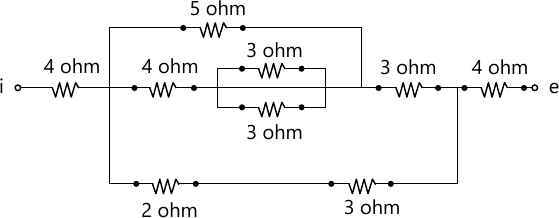3 M
1 (b) State and explain maximum power transfer theorem.
3 M
1 (c) Find the RMS value of current flowing through a 314 ?F capacitor when connected to 230V, 50 Hz, 1-? AC supply.
3 M
1 (d) A series R-L-C circuit is supplied with v(t)=10sin(1000t) volts. If the maximum peak voltage across capacitor is 400 Volts, find the quality factor of the circuit.
3 M
1 (e) Draw and explain the power triangle for a 3-? lagging load.
2 M
1 (f) A 80 kVA, 3200/400V, 1-&Straightphi;, 50 Hz transformer has 111 turns on secondary winding calculate (i) Number of turns on primary winding (ii) Secondary full load current (iii) Cross sectional area of the core if the maximum flux density is 1.2 Tesla.
4 M
1 (g) Justify that full wave rectifier is more efficient that half wave rectifier.
2 M
2 (a) By mesh analysis find the current through 4 ohm resistor for the given circuit shown below.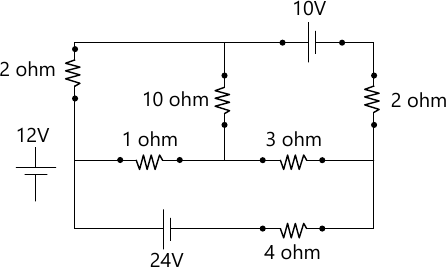6 M
2 (b) When a resistor and a choke coil in series are connected to a 240V supply, a current of 3A flows, lagging 37° behind the supply voltage. while voltage across the coil is 171V. Find resistance of resistor, resistance and reactance of coil.
8 M
2 (c) Draw and explain the phasor diagram for practical transformer connected to lagging power factor load.
6 M
3 (a) A 415V, 50Hz, 3&Straightphi; voltage is applied to three star connected identical impedances each consisting of a resistance of 15 ohm, capacitance of 177 ?F and inductance of 0.1 H in series. Find (i) Phase current (ii) Line Current (iii) power drawn (iv) Power Factor (v) reactive power (vi) Total kVA. Draw phasor diagram.
8 M
3 (b) A 5 kVA, 1000/200V, 50 Hz, 1-&Straighphi;, transformer gave following test results O.C. Test (H.V. Side) : – 100 V, 0.24 A, 90W
S.C. Test (H.V. Side) : – 50 V, 5A, 110W
Calculate
i) Equivalent circuit parameters referred to LV side.
ii) Regulation at Half load at 0.8 lagging power factor.
6 M
3 (c) Explain why we need filter.
2 M
3 (d) Draw and explain the output characteristics of a transistor in CE configuration. Also indicate various regions of operation on the characteristics.
4 M
4 (a) Using source transformation find the current through 5 ohm resistor of network show in fig.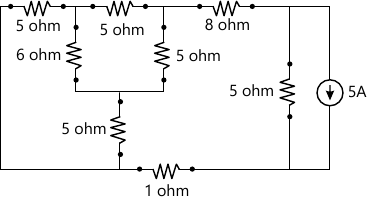7 M
4 (b) Two current i1=100sin (?t+?/4) and i2=25sin (?t-?/6) are fed to common conductor. Find the total current. If the conductor resistance is 10? what is the power dissipated in the conductor.
5 M
4 (c) Explain the measurement of 3&Straightphi; power by two wattmeter method.
4 M
4 (d) Define Ripple factor. What is requirement of a rectifier in terms of ripple factor? How is it achieved?
4 M
5 (a) In the circuit given below find R for maximum power delivered to it. Also find Pmax.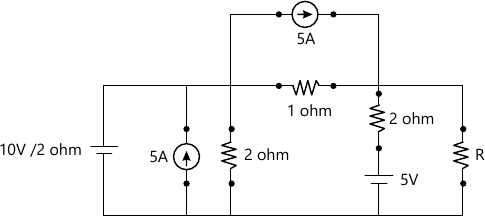8 M
5 (b) Two impedances of 12+j16 ? and 10-J20? are connected in parallel across 230V, 50Hz, 1?, ac supply. Find the kW, kVA, kVAR and power factor of each branch.
4 M
5 (c) Explain transformer test to find the following parameters of transformer
i) Equivalent resistance as referred to primary side
ii) Equivalent core loss resistance
iii) Equivalent leakage reactance as referred to primary side
iv) magnetizing reactance.
8 M
6 (a) By superposition theorem find current through 30 ohm resistor of the network given.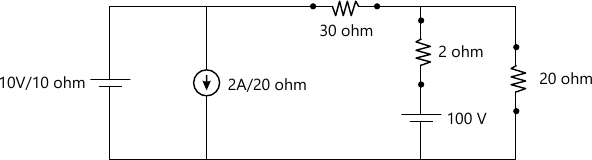7 M
6 (b) A resistance of 20 ohm and a pure inductance of 0.2 H is connected in parallel with 200 ?F capacitor with variable frequency, 230V supply. Find the resonant frequency at which the total current from the supply is in phase with supply voltage. Also find the value of this current.
7 M
6 (c) Each phase of a 3-&Straightphi; delta connected load has an impedance of Zph=50 ? 60 ?. The line voltage is 400V. Calculate total power. What will be the reading of two wattmeters connected to measure the power.
6 M